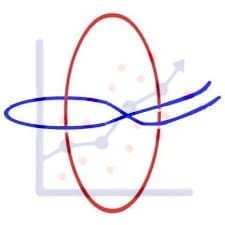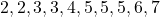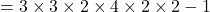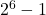Get inspired by the success stories of our students in IIT JAM MS, ISI  MStat, CMI MSc DS.  Learn More

# ISI MStat PSB 2013 Problem 3 | Number of distinct integersThis is a very beautiful sample problem from ISI MStat PSB 2013 Problem 3 based on Counting principle . Let's give it a try !!

## Problem- ISI MStat PSB 2013 Problem 3

1. Suppose integers are formed by taking one or more digits from the
2. following. For example, 355 is a possible choice while 44 is not. Find the number of distinct integers that can be formed in which
3. (a) the digits are non-decreasing;
4. (b) the digits are strictly increasing.

### Prerequisites

Basic Counting Principle

## Solution :

(a) To find the number of integers with non-decreasing digits we will go by this way

First see that the position of given digits are fixed as they have to form non-decreasing digits what can be do is to select number of times the particular digit occurs .

For example 223556 in an integer With non-decreasing digits so here 2 occurs
2 times, 3 occurs 1 times, 4 doesn't occurs any times, 5 occurs 2 times, 6 occur 1 time and finally 7 which doesn't occur any number of times.
So, here we have (0,1,2) possible choices of occurrence of 2 ,(0,1,2) possible choices of occurrence of(0,1) possible choices of occurrence of 7 .

Hence, number of such integers.

We are subtracting 1 to exclude the case where no digits has occur any number of times.

(b) To find the number of integers with increasing digits we can go by above method. Here there is only one restriction that a digit must be greater than it's preceding digits.so, no consecutive digits can be equal. Hence every digits has two choices (0,1) of occurrence . Therefore number of such integers =.

We are subtracting 1 to exclude the case where no digits has occur any number of times.

## Food For Thought

A number is chosen randomly from all the 5 digited numbers.Find out the probability that the digits form a non decreasing sequence.

Hint 1 : Find what is invariant here .

Hint 2 : Use the non-negative integer solutions of a equation formula .

## Subscribe to Cheenta at Youtube

This is a very beautiful sample problem from ISI MStat PSB 2013 Problem 3 based on Counting principle . Let's give it a try !!

## Problem- ISI MStat PSB 2013 Problem 3

1. Suppose integers are formed by taking one or more digits from the
2. following. For example, 355 is a possible choice while 44 is not. Find the number of distinct integers that can be formed in which
3. (a) the digits are non-decreasing;
4. (b) the digits are strictly increasing.

### Prerequisites

Basic Counting Principle

## Solution :

(a) To find the number of integers with non-decreasing digits we will go by this way

First see that the position of given digits are fixed as they have to form non-decreasing digits what can be do is to select number of times the particular digit occurs .

For example 223556 in an integer With non-decreasing digits so here 2 occurs
2 times, 3 occurs 1 times, 4 doesn't occurs any times, 5 occurs 2 times, 6 occur 1 time and finally 7 which doesn't occur any number of times.
So, here we have (0,1,2) possible choices of occurrence of 2 ,(0,1,2) possible choices of occurrence of(0,1) possible choices of occurrence of 7 .

Hence, number of such integers.

We are subtracting 1 to exclude the case where no digits has occur any number of times.

(b) To find the number of integers with increasing digits we can go by above method. Here there is only one restriction that a digit must be greater than it's preceding digits.so, no consecutive digits can be equal. Hence every digits has two choices (0,1) of occurrence . Therefore number of such integers =.

We are subtracting 1 to exclude the case where no digits has occur any number of times.

## Food For Thought

A number is chosen randomly from all the 5 digited numbers.Find out the probability that the digits form a non decreasing sequence.

Hint 1 : Find what is invariant here .

Hint 2 : Use the non-negative integer solutions of a equation formula .

## Subscribe to Cheenta at Youtube

This site uses Akismet to reduce spam. Learn how your comment data is processed.

### Knowledge Partner